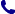• Kontakt
• Newsletter Zapisz się po informacje o nowościach i specjalnych ofertach. Administrator danych: ABE-IPS sp. z o.o.
Wybierz interesujące Cię kategorie
Wyrażam zgodę na przetwarzanie moich danych osobowych w celach marketingowych, tym samym akceptuję Regulamin Newslettera i Politykę prywatności.
Wyrażam zgodę na przesyłanie informacji handlowych drogą elektroniczną.
Wypisz się
• Klienci instytucjonalni
• Dostawa
• O nas
Dostęp on-line

# Książki

0.00 PLN
Schowek (0)
 Schowek jest pusty

## Mass Transfers and Physical Data Estimation

 Autorzy Wydawnictwo ISTE Ltd Data wydania 01/10/2019 Liczba stron 224 Forma publikacji książka w twardej oprawie Poziom zaawansowania Dla profesjonalistów, specjalistów i badaczy naukowych ISBN 9781786302854 Kategorie Termodynamika i ciepło
554.00 PLN (z VAT)
\$147.40 / €123.82 / £110.31 /
Produkt dostępny
Dostawa 2 dni
Ilość
Do schowka

## Opis książki

Many practical operations, such as environment depollution, blood dialysis or product purification, require matter transfer. With an emphasis on the aforementioned subjects, this book revisits the founding principles of materials transfer on the basis of Fick's first law, which constitutes the foundation of diffusional phenomena. Additionally, continuity equations translating the macroscopic balances of systems are established. These balances constitute Fick's second law, which can be applied to quantify the fluxes of matter transferred in each situation, provided physical data is available. To this end, Mass Transfers and Physical Data Estimation pays particular attention to methods of data estimation. Methods presented in this book are applied to several practical cases, such as diffusion in catalytic reactions or the reconstitution of cartilage in human bone joints.

Mass Transfers and Physical Data Estimation

## Spis treści

Preface ix

Introduction xi

Chapter 1. Determination of Physical Data 1

1.1. Introduction 1

1.2. Estimating critical properties 2

1.2.1. Estimating critical temperature 2

1.2.2. Estimating critical pressure 5

1.2.3. Estimating the critical volume: Benson correlation (Benson, 1948) 8

1.2.4. Estimating the critical compressibility factor 10

1.3. Methods for estimating boiling temperature 11

1.4. Methods for estimating density 14

1.4.1. Estimating liquid densities 14

1.5. Methods for estimating viscosity 15

1.5.1. Estimating viscosities of pure liquids 15

1.5.2. Correlations for the viscosity of liquid mixtures 17

1.5.3. Estimating gas viscosities 18

1.6. Methods for estimating specific heat 19

1.6.1. Heat capacities of petroleum oils 19

1.6.2. Heat capacities of petroleum vapors 20

1.6.3. Estimations for anthracite and bituminous coals 20

1.6.4. Heat capacities for cement, mortar and sand 21

1.6.5. Heat capacities of organic liquids 21

1.7. Estimating latent heat of vaporization 22

1.7.1. Rapid estimations 22

1.7.2. Calculating latent heat from critical data 23

1.7.3. Chen correlation 23

1.7.4. Calculations at different temperatures 24

1.8. Estimating expansion coefficients 24

1.9. Methods for estimating heat conductivity 25

1.9.1. Heat conductivity of metals and alloys 25

1.9.2. Heat conductivity of wood 26

1.9.3. Conductivity of chains of liquid hydrocarbons 26

1.9.4. Conductivity of gases and vapors 27

1.9.5. Conductivity of monatomic gases 28

1.9.6. Conductivity of non-polar gases with linear molecules 28

1.10. Physical properties of water 29

1.10.1. Correlation of density 29

1.10.2. Heat capacity 29

1.10.3. Correlation of heat conductivity 29

1.10.4. Correlation of viscosity 29

1.10.5. Correlation of thermal diffusivity 30

1.10.6. Correlation of the Prandtl number 30

1.10.7. Correlation for calculating the expansion coefficient 30

1.10.8. Correlation for calculating the saturating pressure 30

1.10.9. Correlation for calculating latent heat 31

1.11. Physical properties of air 31

1.11.1. Correlation of density 32

1.11.2. Heat capacity 32

1.11.3. Correlation of heat conductivity 32

1.11.4. Correlation of viscosity 33

1.11.5. Correlation of thermal diffusivity 33

1.11.6. Correlation of the Prandtl number 33

1.11.7. Correlation for calculating the expansion coefficient 33

Chapter 2. Determinants and Parameters of Mass Transfer 35

2.1. Introduction 35

2.2. Relative transfer velocities 36

2.2.1. Velocity relating to average mass velocity 36

2.2.2. Velocity relative to average molar velocity. 37

2.3. Amount of matter transferred 38

2.4. Expressions of flux density 39

2.4.1. Total flux 39

2.4.2. Specific fluxes 41

2.5. Operations on diffusion flux densities 44

2.5.1. Total density as a function of the specific densities 44

2.5.2. Sum of mass densities with respect to v 45

2.5.3. Sum of molar flux densities with respect to v* 46

2.5.4. Sum of mass flux densities with respect to a mobile reference frame at v* 46

2.6. Relations between flux densities fi and ji 47

2.7. Relations between flux densities Fi and Ji* 47

Chapter 3. Fick's First Law: Diffusion Coefficients 49

3.1. Introduction 49

3.2. Fick's first law 50

3.2.1. Expressing the flux density vector 50

3.2.2. Similarities to energy and momentum transfer laws 51

3.2.3. Convective analogy 52

3.3. Fick's first law in different forms 52

3.4. Determining diffusion coefficients from tabulated data 53

3.4.1. Gaseous binary diffusion coefficients 53

3.4.2. Illustration: diffusion coefficients of CO2 in air and in water vapor 54

3.4.3. Diffusion coefficients for liquid binaries 58

3.5. Estimating diffusion coefficients from correlations 60

3.5.1. Estimating gaseous binary diffusion coefficients 60

3.5.2. Estimating diffusion coefficients of liquid binaries 71

3.6. Diffusion coefficients for multicomponent mixtures 81

3.6.1. Stefan-Maxwell equation 81

3.6.2. Effective diffusion coefficient for complex mixtures 82

Chapter 4. Fick's Second Law: Macroscopic Balances 85

4.1. Introduction 85

4.2. Overall continuity equation 85

4.2.1. The accumulation term 86

4.2.2. The generation term 86

4.2.3. The term I - O 87

4.2.4. The balance equation 87

4.2.5. The balance equation in Cartesian coordinates 88

4.3. Particular continuity equations 88

4.3.1. The term Ii - Oi 88

4.3.2. The accumulation term 89

4.3.3. The generation term 89

4.3.4. Continuity equations in molar terms 90

4.4. Illustration: diffusion with chemical reaction 92

4.5. Illustration: diffusion of a component in a stagnant mixture 94

4.6. Reading: background to Fick's Laws 97

Chapter 5. Exercises and Solutions 101

Appendices 153

Appendix 1 155

Appendix 2 187

References 191

Index 205

## Polecamy również książki801 777 223